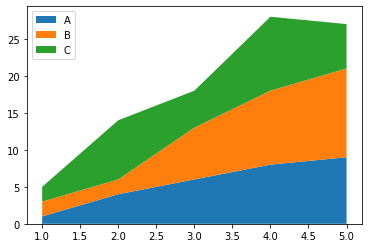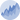# Basic Stacked Area Chart

This post describes how to draw a basic stacked area chart using the matplotlib library of python.

A basic stacked area chart can be plotted by the `stackplot()` function of matplotlib. The parameters passed to the function are:

• `x` : x axis positions
• `y` : y axis positions
• `labels` : labels to assign to each data series

Note that for `y` input, as you can give a sequence of arrays, you can also give multiple arrays. The example below shows both ways.

``````# libraries
import numpy as np
import matplotlib.pyplot as plt

# --- FORMAT 1

# Your x and y axis
x=range(1,6)
y=[ [1,4,6,8,9], [2,2,7,10,12], [2,8,5,10,6] ]

# Basic stacked area chart.
plt.stackplot(x,y, labels=['A','B','C'])
plt.legend(loc='upper left')
plt.show()

# --- FORMAT 2
x=range(1,6)
y1=[1,4,6,8,9]
y2=[2,2,7,10,12]
y3=[2,8,5,10,6]

# Basic stacked area chart.
plt.stackplot(x,y1, y2, y3, labels=['A','B','C'])
plt.legend(loc='upper left')
plt.show()``````## Contact & Edit

👋 This document is a work by Yan Holtz. Any feedback is highly encouraged. You can fill an issue on Github, drop me a message onTwitter, or send an email pasting `yan.holtz.data` with `gmail.com`.